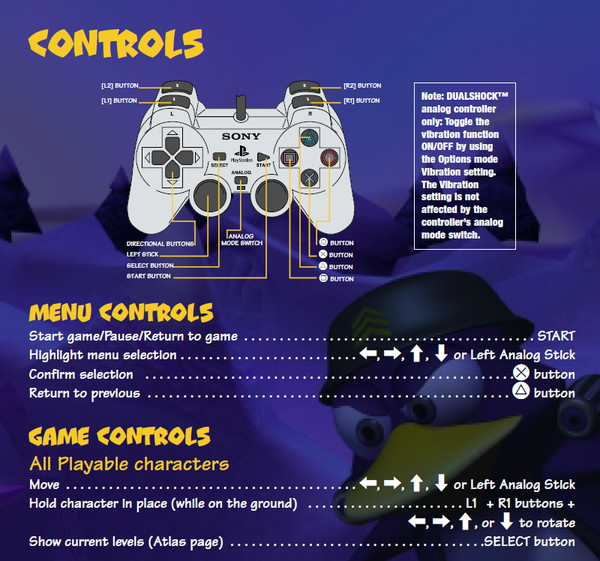# Spyro 3 Game Controls## ControlsSpyro

X = jump
X mid-air = glide
Triangle = hover after glide
X, then triangle = horn smash
Square = charge
Circle = flame

Sheila

X = jump
X mid-air = double jump
X after hitting the ground = jump higher
Circle + square = kick
Triangle mid-air = stomp smash

Sgt. Byrd

X = fly
Hold X = fly higher
Square = drop item
Circle = fire weapon
Hold triangle = aim

Bentley

X = jump
Square = thump
Circle = spin club
Hold triangle = Look around

Agent 9

X = jump
Square = throw bomb
Hold square = aim bomb
Circle = fire weapon
Triangle = enter sniper mode

Report This Post